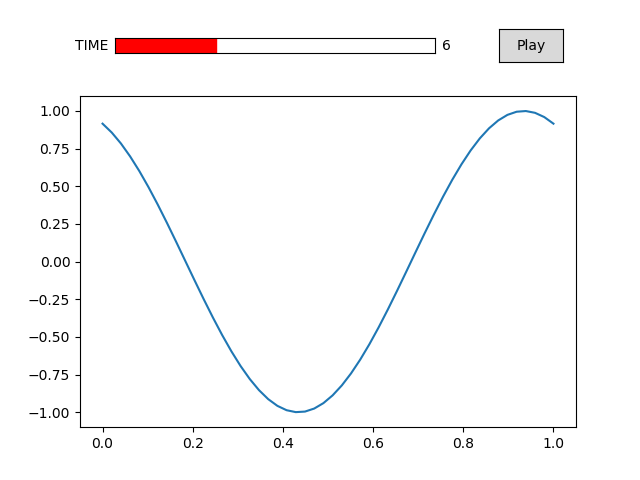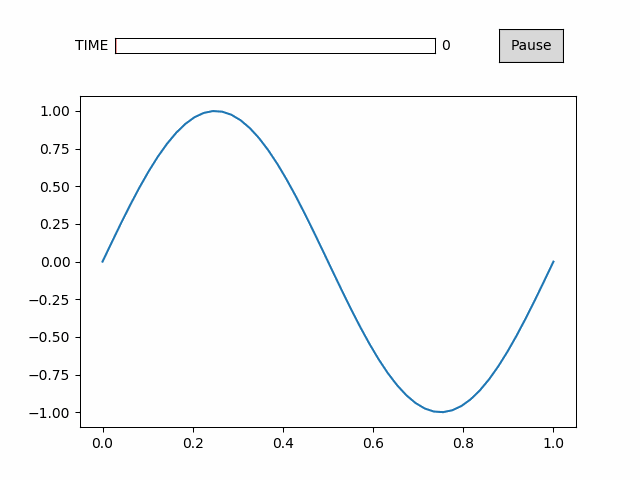# Custimizing the Controls¶

Here we’ll like how to manipulate the timeline_slider and the toggle button.

The interactive controls can be make using the controls() method of the animation class, as in the getting started tutorial, but this method is a wrapper around the toggle and timeline_slider methods.

First, we need from imports and data to animate.

:

%matplotlib notebook
import numpy as np
import matplotlib.pyplot as plt
import animatplot as amp

:

x = np.linspace(0, 1, 50)
t = np.linspace(0, 1, 20)

X, T = np.meshgrid(x, t)
Y = np.sin(2*np.pi*(X+T))

Animation.toggle(ax=None)[source]

Creates a play/pause button to start/stop the animation

Parameters: ax (matplotlib.axes.Axes, optional) – The matplotlib axes to attach the button to.
Animation.timeline_slider(text=’Time’, ax=None, valfmt=None, color=None)[source]

Creates a timeline slider.

Parameters: text (str, optional) – The text to display for the slider. Defaults to ‘Time’ ax (matplotlib.axes.Axes, optional) – The matplotlib axes to attach the slider to. valfmt (str, optional) – a format specifier used to print the time Defaults to ‘%s’ for datetime64, timedelta64 and ‘%1.2f’ otherwise. color – The color of the slider.
Animation.controls(timeline_slider_args={}, toggle_args={})[source]

Creates interactive controls for the animation

Creates both a play/pause button, and a time slider at once

Parameters: timeline_slider_args (Dict, optional) – A dictionary of arguments to be passed to timeline_slider() toggle_args (Dict, optional) – A dictionary of argyments to be passed to toggle()

Now to make the animation

By specifying the ax parameter, we can change the position of either the toggle or the timeline_slider.

We use color to change the color of the slider, and valfmt to change how the time is displayed.

Let’s create our block, then create the controls at the top of the animation.

:

block = amp.blocks.Line(X, Y)

plt.subplots_adjust(top=0.8) # squish the plot to make space for the controls
slider_ax = plt.axes([.18, .89, .5, .03]) # the rect of the axis
button_ax = plt.axes([.78, .87, .1, .07]) # x, y, width, height

anim = amp.Animation([block])

anim.toggle(ax=button_ax)
anim.timeline_slider(text='TIME', ax=slider_ax, color='red', valfmt='%1.0f')
# equivalent to:
# anim.controls({'text':'TIME', 'ax':slider_ax, 'color':'red', 'valfmt':'%1.0f'},
#               {'ax':button_axis})

anim.save_gif('images/controls')
plt.show()